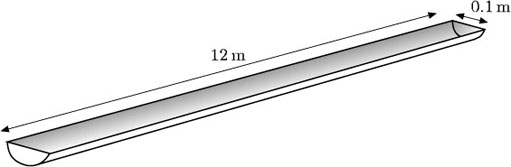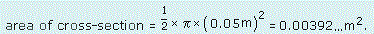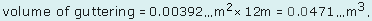Science, Maths & Technology

### Become an OU studentGeometry

Start this free course now. Just create an account and sign in. Enrol and complete the course for a free statement of participation or digital badge if available.

# Try some yourself

## Question 1

Find the volumes of these objects.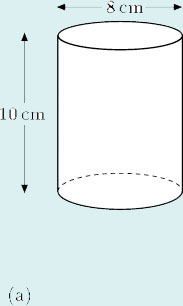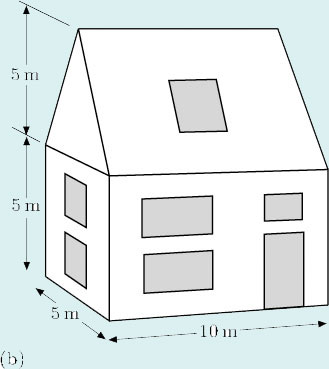(a)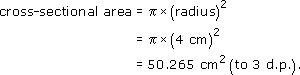So

volume = 50.265 cm2 × 10 cm = 502.65 cm3.

Thus the volume is 503 cm3 (to the nearest cubic centimetre).

(If you used the approximate value of 3.14 for, you will have got a cross-sectional area of 50.24 cm2 and a volume of 502.4 cm3.)

• (b)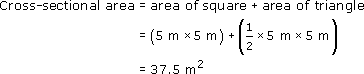So

volume = 37.5 m2 × 10 m = 375 m3.

## Question 2

Two car manufacturers both claim that their models have an engine capacity of 2 litres. The two models have four-cylinder, four-stroke engines.

The table below shows the details of the four cylinders.

Car modelCylinder diameter (bore)/mmCylinder height (stroke)/mmNumber of cylinders
A86864
B92754

By working out the total volume of the four cylinders for each model in cm3, find out if the manufacturers’ claims are true.

(Hint: 1 litre = 1000 cm3.)

Car A has four cylinders, each with a radius of 4.3 cm and a height of 8.6 cm. The volume of one cylinder is calculated by using the formula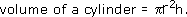So, the four cylinders will have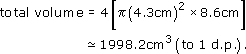Car B has four cylinders, each with a radius of 4.6 cm and a height of 7.5 cm. From the same formula, the four cylinders will have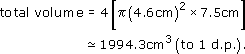Therefore, both engines have a cubic capacity very close to 2000 cm3. They are both said to have two-litre engines. Hence the claims of both manufacturers are true.

## Question 3

The guttering pictured here has a semicircular cross-section. Find the volume of water that the guttering will hold when full.